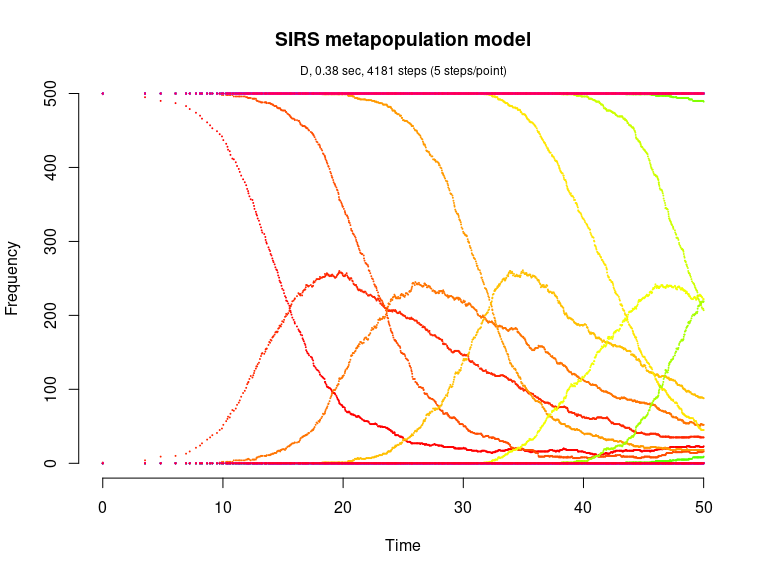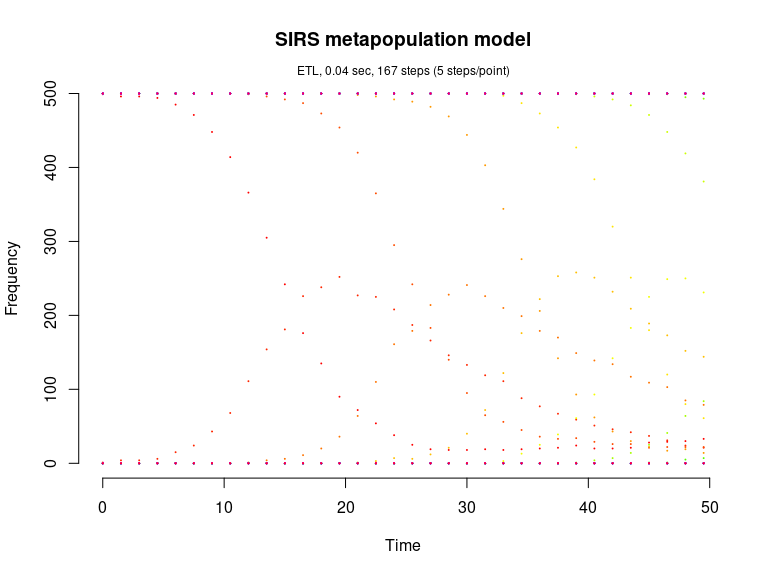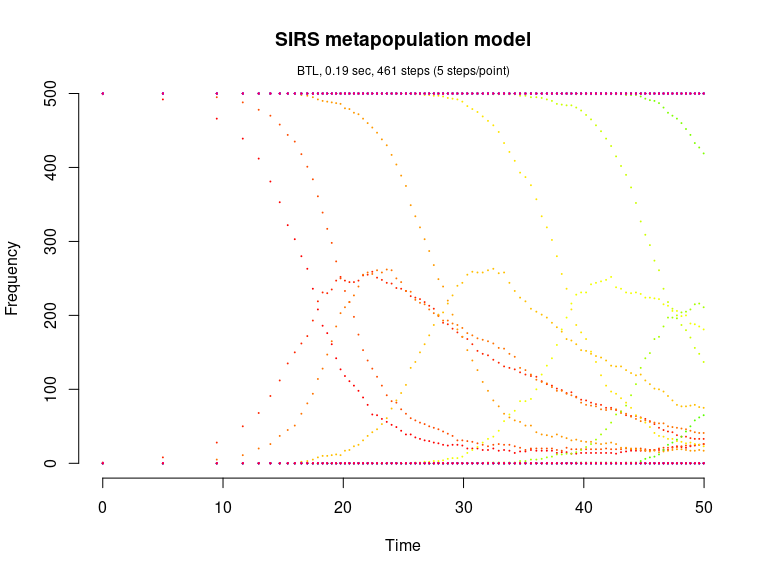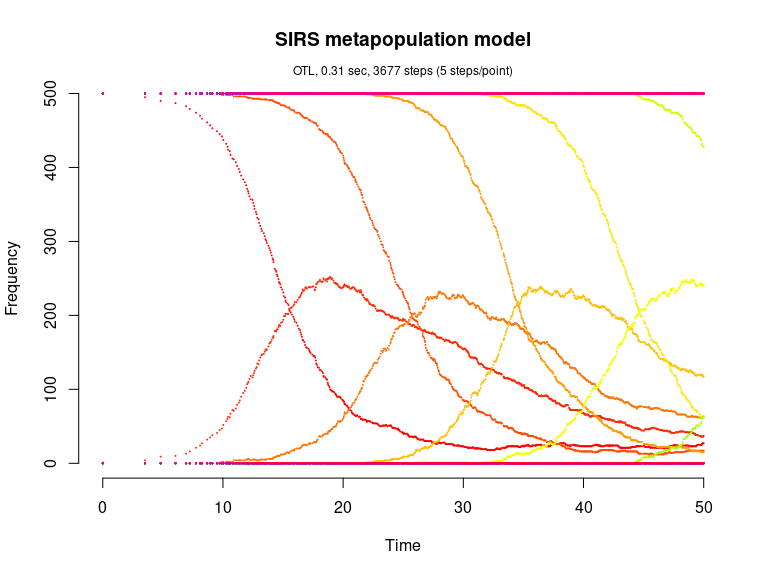# SIRS metapopulation model (Pineda-Krch, 2008)

The SIRS epidemiological metapopulation model is defined in Pineda-Krch (2008).

library(GillespieSSA)

Define parameters

patchPopSize <- 500                    # Patch size
U <- 20                                # Number of patches
simName <- "SIRS metapopulation model" # Simulation name
tf <- 50                               # Final time

parms <- c(
beta = 0.001,                        # Transmission rate
gamma = 0.1,                         # Recovery rate
rho = 0.005,                         # Loss of immunity rate
epsilon = 0.01,                      # Proportion inter-patch transmissions
N = patchPopSize                     # Patch population size (constant)
) 

Create the named initial state vector for the U-patch system. The structure of x0 is as follows (assuming a patchsize of 500 individuals),

x0 <- c(
S1 = 499, I1 = 1,
S2 = 500, I2 = 0,
S3 = 500, I3 = 0,
...
S20 = 500, I20 = 0
)
x0 <- c(patchPopSize - 1, 1, rep(c(patchPopSize, 0), U - 1))
names(x0) <- unlist(lapply(seq_len(U), function(i) paste0(c("S", "I"), i)))

Define the state change matrix for a single patch

nu <- matrix(c(-1, -1,  0, +1,  # S
+1, +1, -1,  0), # I
nrow=2,byrow=TRUE)

Define propensity functions

a <- unlist(lapply(
seq_len(U),
function(patch) {
i <- patch
j <- if (patch == 1) U else patch - 1

c(
paste0("(1-epsilon)*beta*S", i, "*I", i), # Intra-patch infection
paste0("epsilon*beta*S", i, "*I", j),     # Inter-patch infection
paste0("gamma*I", i),                     # Recovery from infection
paste0("rho*(N-S", i, "-I", i, ")")       # Loss of immunity
)
}
))

Run simulations with the Direct method

set.seed(1)
out <- ssa(
x0 = x0,
a = a,
nu = nu,
parms = parms,
tf = tf,
method = ssa.d(),
simName = simName,
verbose = FALSE,
consoleInterval = 1
)
ssa.plot(out, by = 5, show.title = TRUE, show.legend = FALSE)Run simulations with the Explict tau-leap method

set.seed(1)
out <- ssa(
x0 = x0,
a = a,
nu = nu,
parms = parms,
tf = tf,
method = ssa.etl(),
simName = simName,
verbose = FALSE,
consoleInterval = 1
)
ssa.plot(out, by = 5, show.title = TRUE, show.legend = FALSE)Run simulations with the Binomial tau-leap method

set.seed(1)
out <- ssa(
x0 = x0,
a = a,
nu = nu,
parms = parms,
tf = tf,
method = ssa.btl(),
simName = simName,
verbose = FALSE,
consoleInterval = 1
)
ssa.plot(out, by = 5, show.title = TRUE, show.legend = FALSE)Run simulations with the Optimized tau-leap method

set.seed(1)
out <- ssa(
x0 = x0,
a = a,
nu = nu,
parms = parms,
tf = tf,
method = ssa.otl(hor = rep(2, length(x0))),
simName = simName,
verbose = FALSE,
consoleInterval = 1
)
ssa.plot(out, by = 5, show.title = TRUE, show.legend = FALSE)